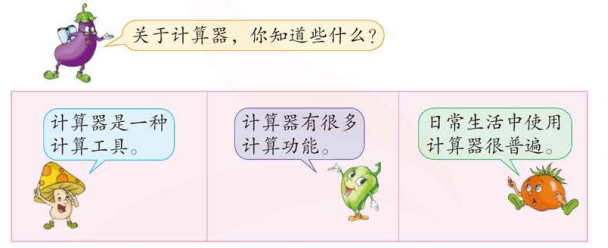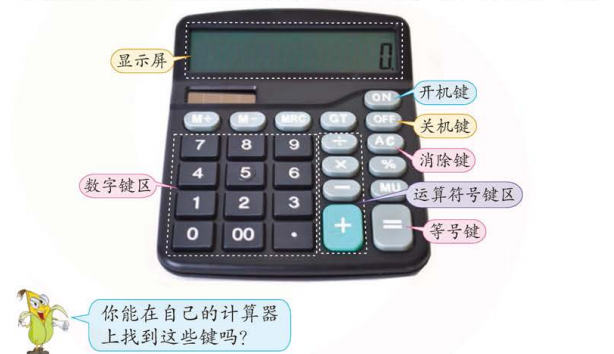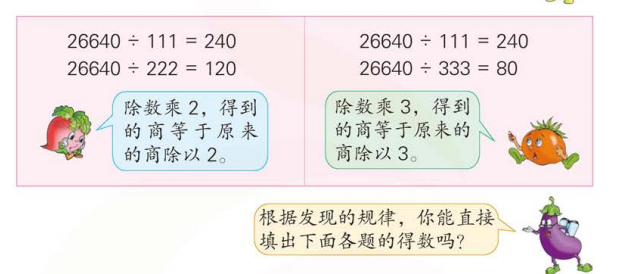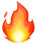## 苏教版数学四年级下册电子课本教材1、用计算器计算38+27、30×18

38+27=

30x18=

2、用计算器计算 40000-165x1820

40000-165x182

=40000-______

=________

3、用26640分别除以111、222和333,商各是多少？用计算器算一算。

26640÷111=    26640÷222=   26640÷333=26640÷444=                        26640÷555=

26640÷666=                       26640÷888=